Courses

# Boundary Layer Theory Civil Engineering (CE) Notes | EduRev

## Civil Engineering (CE) : Boundary Layer Theory Civil Engineering (CE) Notes | EduRev

The document Boundary Layer Theory Civil Engineering (CE) Notes | EduRev is a part of the Civil Engineering (CE) Course Civil Engineering SSC JE (Technical).
All you need of Civil Engineering (CE) at this link: Civil Engineering (CE)

Chapter 9 Boundary Layer Theory

•  The concept of boundary layer was first introduced by L. Prandt in 1904.
• Boundary layer is a region in the immediate vicinity of the boundary surface in which the velocity of flowing fluid increases gradually from zero at the boundary surface to the velocity of the main stream.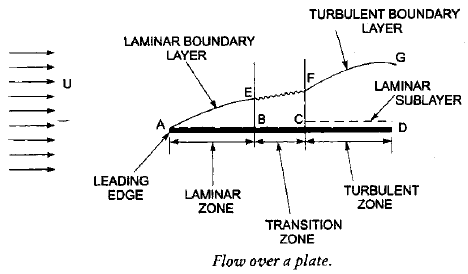•  Boundary Conditions of Boundary Layer Region

(i) at y = 0, v = 0
(ii) at y = d, v = V0 (d = boundary layer thickness V0 = free stream velocity)

(iii) at y = d, dv/dy   = 0 [∵ v = V0 = constant]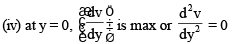•  Boundary layer thickness (d) It is defined as the distance from the boundary surface in which the velocity reaches the 99% of the velocity of the main stream.
y =d for v = 0.99 V0

• Displacement Thickness (d*) It is the distance measured normal to the boundary, by which the free stream is displaced on account of the formation of boundary layer.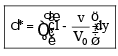The quantity (V– v) is known as the velocity defect.

• Momentum Thickness (q)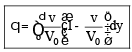The ratio of displacement thickness to momentum thickness is called the shape factor (H)

H = d/q

•   Its value should always greater than 1
• Energy Thickness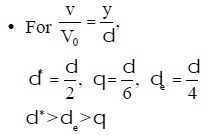•   Von-Korman momentum integral equation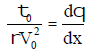• Blassius experimental results/ when
• Laminar Conditions : Velocity distribution is parabolic

(i) Boundary Layer thickness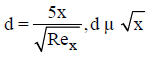(ii) Local coefficient of drag C*D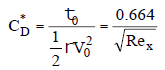(iii) Avg. Coefficient CD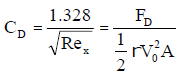•   Turbulent Conditions : Velocity distributions is logarithmic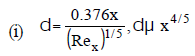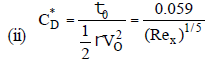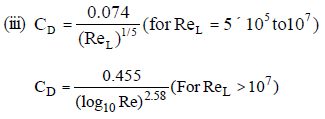where
d = Boundary layer thickness
t = Shear stress at solid surface
x = Distance from where solid surface starts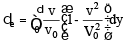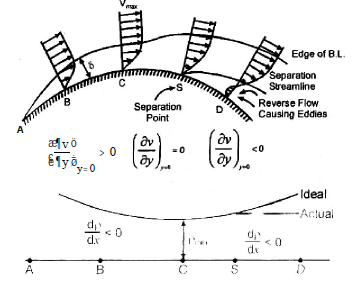• Location of Separation point : The separation point S is determined from the condition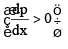For a given velocity it can be determined whether the B.L.(Boundary layer) has separated or on the verge of separation or will not separate from the following conditions :
1. If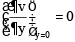< 0 : Flow has separated.
2. If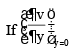= 0 : Flow is on verge of separation.
3. If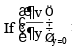> 0 : Flow is attached with the surface

• Methods of Preventing Separation

(i) Rotating the boundary in the direction of flow.
(ii) Suction of the slow moving fluid by a suction slot.
(iii) Supplying additional energy from a blower.
(iv) Providing a bypass in the slotted wing.
(v) Providing guide blades in a bend.
(vi) Injecting fluid into boundary layer.
(vii)Streamlining of body shapes.

Offer running on EduRev: Apply code STAYHOME200 to get INR 200 off on our premium plan EduRev Infinity!

## Civil Engineering SSC JE (Technical)

109 docs|50 tests

,

,

,

,

,

,

,

,

,

,

,

,

,

,

,

,

,

,

,

,

,

;